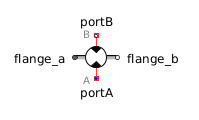Transfer Function - MapleSim Help

Transfer Function

Single Input Single Output continuous control blockDescription The Transfer Function component multiplies the complex input by a transfer function consisting of a rational polynomial in $s$ specified by the real vector parameters $a$ and $b$ and evaluated at $s=jw$, where $w$ is a real input.Equations $y=\frac{\sum _{k=1}^{{n}_{b}}{b}_{k}{\left(jw\right)}^{{n}_{b}-1}}{\sum _{k=1}^{{n}_{a}}{a}_{k}{\left(jw\right)}^{{n}_{a}-1}}\left\{\begin{array}{cc}\stackrel{&conjugate0;}{u}& \mathrm{Use Conjugate Input}\\ u& \mathrm{otherwise}\end{array}$ ${n}_{a}=\mathrm{dimension}\left(a\right)$ ${n}_{b}=\mathrm{dimension}\left(b\right)$Connections

 Name Description Modelica ID $u$ Complex input signal u $y$ Complex output signal y $w$ Real input; angular frequency input in $\frac{\mathrm{rad}}{s}$ wParameters

 Name Default Units Description Modelica ID Use Conjugate Input $\mathrm{false}$ If true, use the complex conjugate of the input useConjugateInput $b$ $\left[1\right]$ Numerator coefficients of transfer function (for example, 2s+3 is specified as {2,3}) b $a$ $\left[1\right]$ Denominator coefficients of transfer function (for example, 5s+6 is specified as {5,6}) aModelica Standard Library The component described in this topic is from the Modelica Standard Library. To view the original documentation, which includes author and copyright information, click here.